# Simple Linear Regression in Machine Learning

In this page we will learn about What is Simple Linear Regression in Machine Learning?, Simple Linear Regression Model, Data Pre-processing, Fitting the Simple Linear Regression to the Training Set, Prediction of test set result, visualizing the Training set results, visualizing the Test set results.

## What is Simple Linear Regression in Machine Learning?

A type of regression technique known as simple linear regression models the relationship between a dependent variable and a single independent variable. A Simple Linear Regression model shows a linear or sloped straight line relationship, which is why it is called Simple Linear Regression.

The dependant variable must be a continuous/real value, which is the most important aspect of Simple Linear Regression. The independent variable, on the other hand, can be assessed using either continuous or categorical values.

The major goals of the simple linear regression algorithm are:

• Create a model that depicts the link between the two variables. Such as the income-to-expenditure ratio, experience-to-salary ratio, and so on.
• New observations are being predicted. For example, weather predicting based on temperature, corporation revenue based on annual investments, and so on.

## Simple Linear Regression Model:

The Simple Linear Regression model can be represented using the below equation:

### y = a0 + a1x+ ε

Where,

a0 = It is the intercept of the Regression line (can be obtained putting x=0)

a1 = It is the slope of the regression line, which tells whether the line is increasing or decreasing.

ε = The error term. (For a good model it will be negligible)

Implementation of Simple Linear Regression Algorithm using Python
Problem Statement example for Simple Linear Regression:

• We're looking to see if there's a link between these two variables.
• The best fit line for the dataset will be found.
• Changes in the dependent variable as a result of the independent variable.

In this step, we'll build a Simple Linear Regression model to determine which line best represents the relationship between these two variables.

To use Python to create the Simple Linear regression model in machine learning, follow the steps below:

## Step-1: Data Pre-processing

Data pre-processing is the initial stage in developing the Simple Linear Regression model. It's something we've already done in this tutorial. However, there will be certain adjustments, which are outlined in the stages below:

• To begin, we'll import three key libraries that will aid us in loading the dataset, plotting graphs, and building the Simple Linear Regression model.

``````
import numpy as np
import matplotlib.pyplot as mtp
import pandas as pd
```
```
• Next, we will load the dataset into our code:

``````
```
```

By executing the above line of code (ctrl+ENTER), we can read the dataset on our Spyder IDE screen by clicking on the variable explorer option.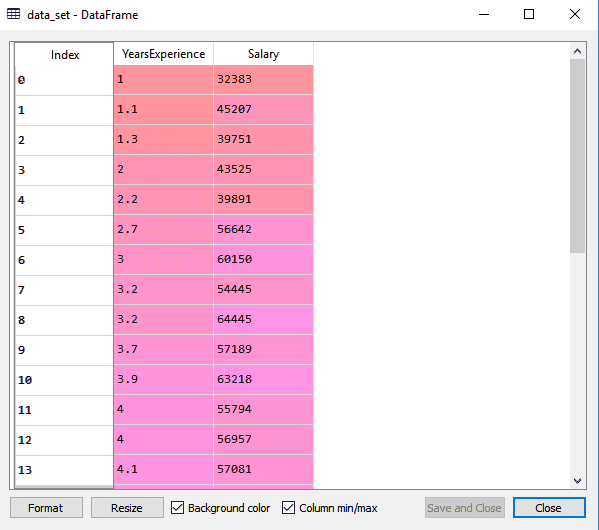The dataset, which has two variables: salary and experience, is shown in the result above.

Note: The folder containing the code file must be saved as a working directory in Spyder IDE, and the dataset or csv file must be in the same folder.

• The dependent and independent variables must then be extracted from the given dataset. Years of experience is the independent variable, and salary is the dependent variable. The following is the code for it:

``````
x = data_set.iloc[:, :-1].values
y = data_set.iloc[:, 1].values
```
```

We used a -1 value for the x variable in the preceding lines of code because we want to eliminate the last column from the dataset. We used 1 as a parameter for the y variable because we want to extract the second column and indexing starts at zero.

If we run the above piece of code, we will obtain the following results for the X and Y variables: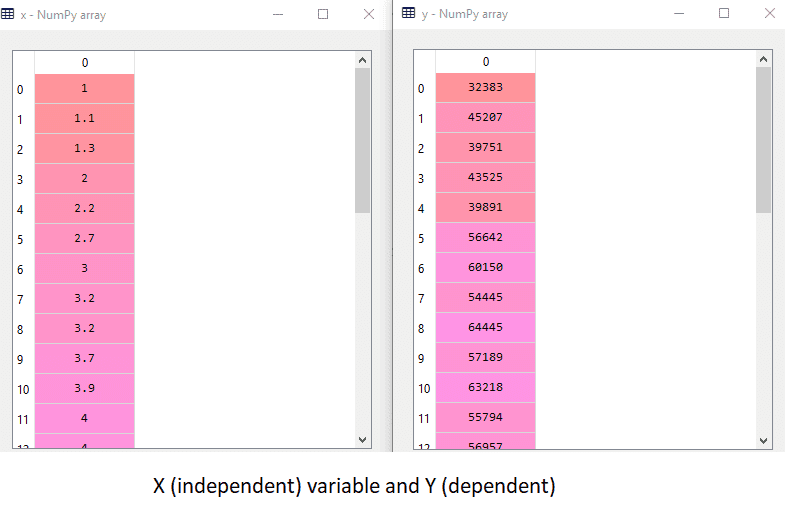The X (independent) variable and Y (dependent) variable have been retrieved from the given dataset, as shown in the above output image.

• After that, we'll divide both variables into test and training sets. Because we have 30 observations, we'll use 20 for the training set and 10 for the test set. We've divided our dataset into two parts so that we can train our model with one and then test it with the other. The following is the code for this:
``````
#Splitting the dataset into training and test set.
from sklearn.model_selection import train_test_split
x_train, x_test, y_train, y_test= train_test_split(x, y, test_size = 1/3, random_state = 0)
```
```

We will get x-test, x-train and y-test, y-train dataset by executing the above code. Consider the below images:

### Test Dataset: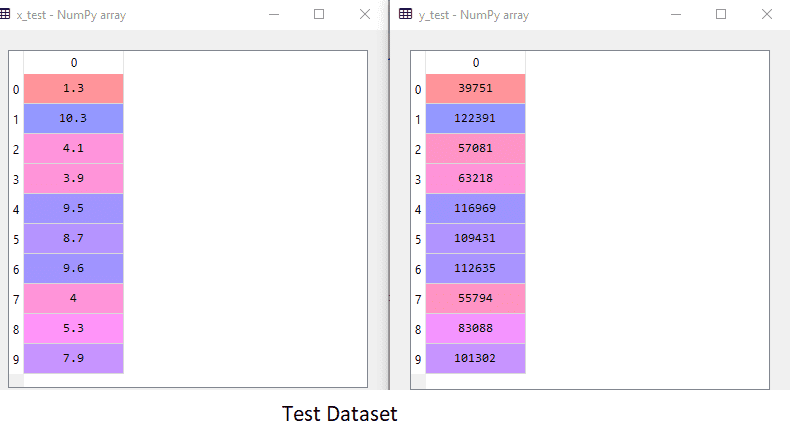### Training Dataset:

When we provide the input values (data) to the function, it gives the S-curve as follows: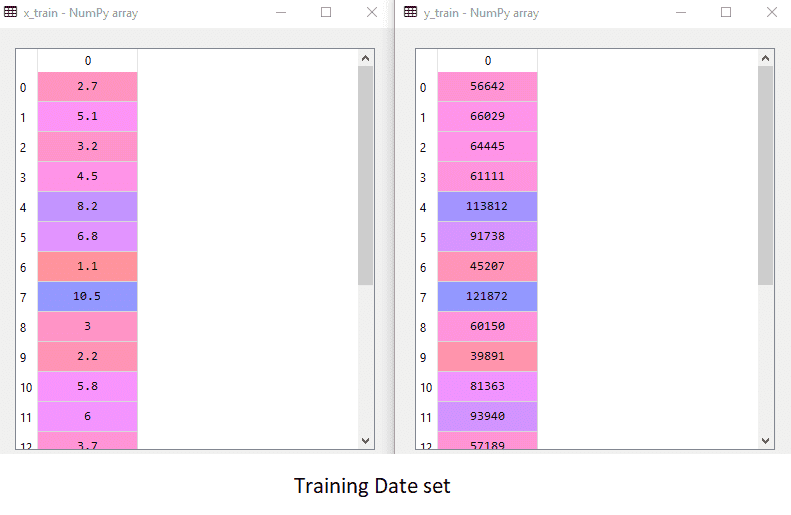• We shall not employ Feature Scaling in simple linear regression. We don't need to do that here because Python libraries take care of it in some circumstances. Now that our information is ready to work with, we'll begin developing a Simple Linear Regression model to solve the problem.

## Step-2: Fitting the Simple Linear Regression to the Training Set:

Fitting our model to the training dataset is the next step. To accomplish so, we'll use the Linear-model library's Linear Regression class from scikit learn. Following the import of the class, we'll create a regressor object. The following is the code for this:

``````
#Fitting the Simple Linear Regression model to the training dataset
from sklearn.linear_model import LinearRegression
regressor = LinearRegression()
regressor.fit(x_train, y_train)
```
```

We used the fit() function to fit our Simple Linear Regression object to the training set in the previous code. We gave the x_train and y_train parameters to the fit() method, which are our training datasets for the dependent and independent variables, respectively. Our regressor object has been fitted to the training set so that the model can learn the correlations between the predictor and target variables quickly. We will obtain the following output after executing the above lines of code.

## Output:

``````
Out: LinearRegression(copy_X=True, fit_intercept=True, n_jobs=None, normalize=False)
```
```

## Step: 3. Prediction of test set result:

Salary is a dependent variable, and salary is an independent variable (Experience). As a result, our model is now ready to forecast the results of the new observations. In this stage, we'll give the model the test dataset (new observations) to see if it can correctly predict the outcome.

We'll make two prediction vectors, y_pred and x_pred, which will include test dataset and training set predictions, respectively.

``````
#Prediction of Test and Training set result
y_pred = regressor.predict(x_test)
x_pred = regressor.predict(x_train)
```
```

When the above lines of code are run, two variables named y_pred and x_pred will appear in the variable explorer options, containing salary forecasts for the training and test sets, respectively.

Output:

You may inspect the variable by using the IDE's variable explorer tool, and you can compare the results by comparing y_pred and y_test values. We can see how well our model is functioning by comparing these numbers.

## Step: 4. visualizing the Training set results:

We will now visualize the training set result in this phase. We'll perform this with the scatter() function from the pyplot package, which we already imported during the pre-processing stage. The scatter () function plots observations in a scatter plot.

Employees' Years of Experience will be plotted on the x-axis, and their salaries will be plotted on the y-axis. The real values of the training set, which are a year of experience x_train, a training set of Salaries y_train, and the color of the observations, will be passed to the function. We're going to use green as our observation color, but you can use any color you want.

Now we need to plot the regression line, so we'll use the pyplot library's plot() function for that. We'll send the years of experience for the training set, the expected pay for the training set x_pred, and the line's color to this function.

The plot's title will be given next. So, we'll use the pyplot library's title() function and supply the term ("Salary vs Experience (Training Dataset)").

After that, we'll use the xlabel() and ylabel() functions to give labels to the x- and y-axes, respectively. Finally, we will use display to represent all of the above on a graph (). The code is as follows:

``````
mtp.scatter(x_train, y_train, color = "green")
mtp.plot(x_train, x_pred, color = "red")
mtp.title("Salary vs Experience (Training Dataset)")
mtp.xlabel("Years of Experience")
mtp.ylabel("Salary(In Rupees)")
mtp.show()
```
```

### Output

By executing the above lines of code, we will get the below graph plot as an output.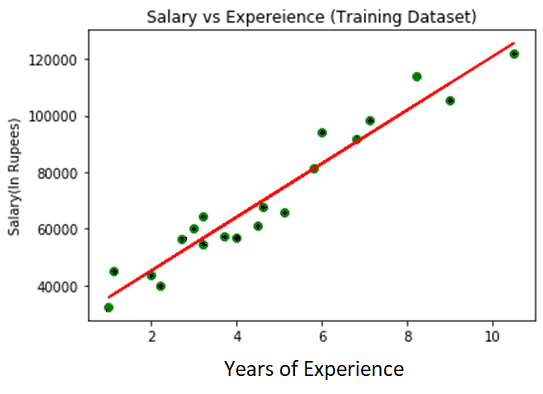The true values observations are shown in green dots in the above image, while anticipated values are covered by the red regression line. A correlation exists between the dependent and independent variables, as shown by the regression line.

Calculating the difference between real and projected values might reveal how well the line fits. However, as seen in the graph above, the majority of the observations are close to the regression line, indicating that our model is suitable for the training set.

## Step: 5. visualizing the Test set results:

We visualized our model's performance on the training set in the previous stage. We'll now repeat the process for the Test set. The entire code will be the same as before, except that instead of x_train and y _rain, we will use x test and y test.

To distinguish the two plots, we change the color of the observations and regression line, but this is optional.

``````
#visualizing the Test set results
mtp.scatter(x_test, y_test, color = "blue")
mtp.plot(x_train, x_pred, color = "red")
mtp.title("Salary vs Experience (Test Dataset)")
mtp.xlabel("Years of Experience")
mtp.ylabel("Salary(In Rupees)")
mtp.show()
```
```

### Output

By executing the above line of code, we will get the output as: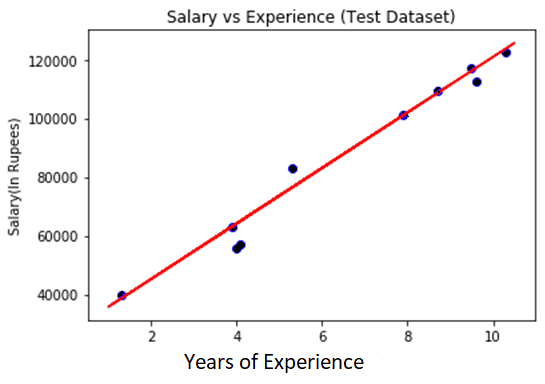The blue hue represents observations, and the red regression line represents prediction in the above plot. As can be seen, the majority of the observations are close to the regression line, indicating that our Simple Linear Regression is a good model capable of making accurate predictions.